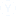# How to improve my calculations?

Can someone please suggest me some easy ways to make my calculations quicker and more accurate?

• Vivek
• 31 oct.
• 1208 Visto
• 2 Respuestas

Improve Calculation Skill in 7 Steps:

There are some easy step, by following which you can improve your calculation skills:

First, start with small and easy calculation like 300+550 =850, small multiplication and easy division etc. Do not start solving complicated calculation directly. Even if you know initial addition, subtraction, multiplication and division, then try to solve them quickly as much as you can.

2. Learn Tricks For Large Multiplication Or Division:
While doing large multiplication you can make use of tables or patterns, which make your calculation less time consuming and easy. Practice this pattern on table until you feel that you are perfect and can use it randomly at any time.
Some more easy tricks for division are as follows:
i) When number is divide by 2: if the last digit of the number is even number then is divide by 2.
ii) When number is divide by 4 - If the last two digits of the number is divide by 4, then the number is divisible by 4.
For example, we have 15237716 then 16 ÷ 4 = 4.
iii) When divide by 5 - If the number ends with the 5 or 0 then that number is divide by 5.
iv)When number is divide by 9 - if the sum of all the digits in the number is divisible by 9, then the number is divisible by 9.
For example, we have 183321477 is divisible by 9
=>1+8+3+3+2+1+4+7+7 = 36 and 36 ÷ 9 = 4.
Same rule is use for the number 3.

3. Write All Calculation:
Whether you do your calculation in your mind or use some trick always try to write all the calculation. It makes your problem easier.

4. Can Make Use Of Your Finger:
Calculating in your finger is an old trick, but still its very useful. First, you have to learn how to count all the numbers on your fingers and then make use this to calculate the numbers.

5. Game:
Gaming is the best way to improve calculation skill. Game like chess in which it all comes down to the decisions you make based on the number of moves you can think ahead. Chess is all about the calculation you make in your head.

6. Practice:
You might have seen your math helper they hardly use calculator for calculation. This is because of the practice. Practice makes you perfect.

7. Internet:
Internet is the source for everything so it is best for improving your calculation skill too. Many free online math tutors are available you can ask them for the interesting calculation tricks.

Hope it helps!Otras discusiones relacionadasPractica simulacro de prueba para
aptitude